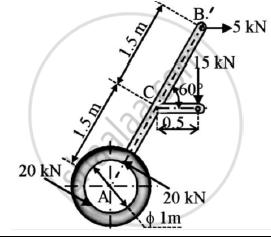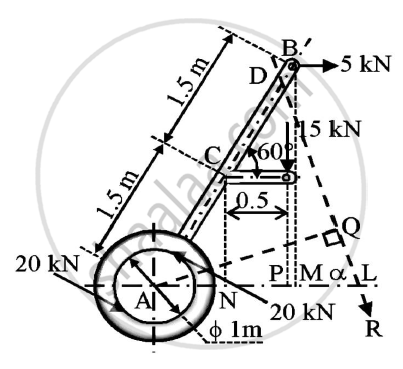# A Machine Part is Subjected to Forces as Shown.Find the Resultant of Forces in Magnitude and in Direction. Also Locate the Point Where Resultant Cuts the Centre Line of Bar Ab. - Engineering Mechanics

A machine part is subjected to forces as shown.Find the resultant of forces in magnitude and in direction.
Also locate the point where resultant cuts the centre line of bar AB.Solution:
Given : A machine subjected to various forces
To find : Resultant of forces
Point where the resultant force cuts the bar AB

#### SolutionIn △BAM,

∠A=60°
AB=3 m
BM=3sin60
=2.5981 m

In △CAN
AC=1.5m
AN=1.5cos60
=0.75 m
AP=AN+NP
=0.75+0.5
=1.25 m

Two 20N forces are equal and opposite in direction.Hence,they form a couple
Perpendicular distance between two 20 N forces = 1m
Moment of couple = 20 x 1
=20 kN-m (Anti clockwise)
Assume R is the resultant of the forces and it is inclined at an angle θ with horizontal

ΣF_X=5 kN

ΣF_Y=-15 kN
R=sqrt( F_x^2)+ (F_Y^2)
=sqrt(5^2 +(−15)^2)
=15.8114 kN

θ = tan^(-1)((R_y)/(R_X))
=tan^(-1)((−15)/5)

Assume that the resultant cut the center line of bar AB at point D
Applying Varigon’s theorem

ΣM_A=ΣM_A^R
-5 x BM -15 x AP +20 = R x AQ
-11.7405 = -15.8114 x AQ
AQ = 0.7425 m

In △AQL, ∠ALQ=θ

∠QAL=90 - θ

∠BAL=60

= θ - 30
=71.5651 - 30

=41.5651°

In△DAQ,cos QAD= (AQ)/(AD)
AD=(AQ)/(cosDAQ) = (0.7425)/(cos41.5651) = 0.9924 m

Resultant force = 15.8114 kN(at 71.5651° in fourth quadrant)
It cuts the center line of bar AB at point D such that AD=0.9924m

Concept: Varignon’s Theorem
Is there an error in this question or solution?